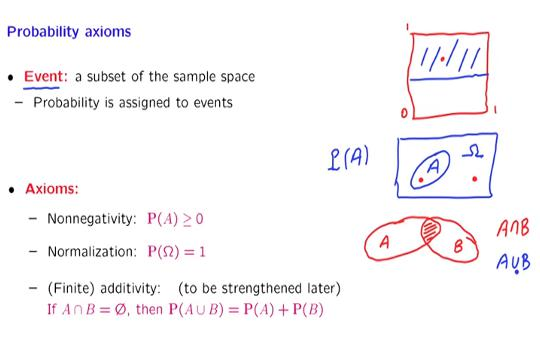# InfoCoBuild

## Res.6-012 Introduction to Probability

Res.6-012 Introduction to Probability (Spring 2018, MIT OCW). Instructors: Prof. John Tsitsiklis and Prof. Patrick Jaillet. The tools of probability theory, and of the related field of statistical inference, are the keys for being able to analyze and make sense of data. These tools underlie important advances in many fields, from the basic sciences to engineering and management. This resource is a companion site to 6.041SC Probabilistic Systems Analysis and Applied Probability. (from ocw.mit.edu)Part I: The Fundamentals Lecture 01 - Probability Models and Axioms Lecture s01 - Supplement: Mathematical Background Lecture 02 - Conditioning and Bayes' Rule Lecture 03 - Independence Lecture 04 - Counting Lecture 05 - Discrete Random Variables, Part I Lecture 06 - Discrete Random Variables, Part II Lecture 07 - Discrete Random Variables, Part III Lecture 08 - Continuous Random Variables, Part I Lecture 09 - Continuous Random Variables, Part II Lecture 10 - Continuous Random Variables, Part III Lecture 11 - Derived Distributions Lecture 12 - Sum of Independent Random Variables Covariance and Correlation Lecture 13 - Conditional Expectation and Variance Revisited Part II: Inference and Limit Theorems Lecture 14 - Introduction to Bayesian Inference Lecture 15 - Linear Modes and Normal Noise Lecture 16 - Least Mean Squares (LMS) Estimation Lecture 17 - Linear Least Mean Squares (LLMS) Estimation Lecture 18 - Inequalities, Convergence, and the Weak Law of Large Numbers Lecture 19 - The Central Limit Theorem (CLT) Lecture 20 - An Introduction to Classical Statistics Part III: Random Processes Lecture 21 - The Bernoulli Process Lecture 22 - The Poisson Process, Part I Lecture 23 - The Poisson Process, Part II Lecture 24 - Finite-State Markov Chains Lecture 25 - Steady-State Behavior of Markov Chains Lecture 26 - Absorption Probabilities and Expected Time to Absorption

 References Res.6-012 Introduction to Probability Instructors: Prof. John Tsitsiklis and Prof. Patrick Jaillet. The tools of probability theory, and of the related field of statistical inference, are the keys for being able to analyze and make sense of data.